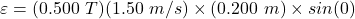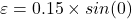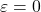A 0.200 m wire is moved parallel to a 0.500 T magnetic field at a speed of 1.50 m/s. What emf is induced across the ends of the

Question

A 0.200 m wire is moved parallel to a 0.500 T
magnetic field at a speed of 1.50 m/s. What emf is
induced across the ends of the wire?

in progress 0
6 months 2021-07-21T15:42:28+00:00 1 Answers 69 views 0

The required emf moved across the wire is zero

Explanation:

For a moving charge particle, the magnetic force can be determined by using the formula;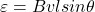since the wire moves in parallel, the angle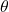between magnetic field and velocity = 0°

B = 0.500 T

v = 1.50 m/s

l = 0.200 m